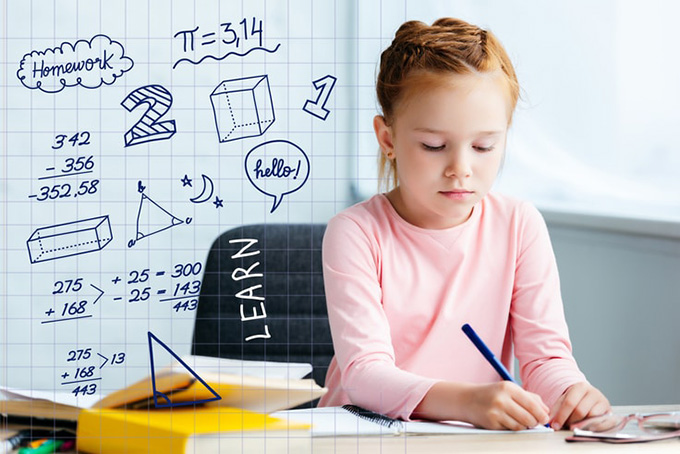# ‘Why would they change maths?’ How your child’s maths education might be very different from yours

Share:There is a scene in the film Incredibles 2 where young Dash asks his dad Bob for help with his maths homework. Bob obliges and begins to scribble on a notepad. But Dash quickly points to his textbook and says, “that’s not the way you’re supposed to do it, Dad”.

Frustrated, Bob exclaims
I don’t know that way! Why would they change math? Math is math!

Many parents trying to help their children with maths may be asking the same sort of question.

Pythagoras’ Theorem is as accurate today as it was when it was discovered millennia ago, and it will continue to remain so. But teachers today also teach maths very differently from when parents were at school.

#### Mental connections not procedures

The teaching and learning of maths has undergone a transformation in past 30 years.

In the past there has been a focus on teaching students procedures, such as times tables and how to work out the circumference of a circle or solve an equation.

We now appreciate the importance of forming mental connections between concepts. For example, when students understand the connection between similar triangles and trigonometry they understand the definition of trigonometric ratios at a much deeper level.

This is because we know today’s learners need to be able to transfer their mathematical understanding to complex, unfamiliar situations.

This means they need to be able to do more than apply a formula and get an answer right. They need to be able to solve problems as they arise.

#### Problem solving and reasoning

Today, the shift in mathematics education is reflected in key mathematical proficiencies in the Australian school curriculum. These include:

• understanding mathematical concepts and procedures
• being fluent in applying mathematical concepts efficiently and accurately
• drawing on mathematical skills and knowledge to solve challenging questions where solutions are not immediately obvious, and
• developing skills in logical thought, and justifying the use of strategies and conclusions reached.

#### Encouraging inventiveness

One practical way teachers are developing students’ mathematical proficiency is by encouraging students to be inventive in the way they solve mathematical problems.

They are deliberately showing students different and multiple ways to represent mathematics problems to give students the space to develop understanding. This also give them an opportunity to reason, model and engage in mathematical thinking.

For example, your child might bring home problems to solve using the area model for multiplication, which looks quite different to a traditional method. For example, we teach how 8×27 can be modelled in parts – 8×20 and 8×7.

When adding numbers, we teach students to deeply understand the place value of each number. Doing so makes our calculations efficient and supports the development of mental strategies for computation.

For example, to add 27 and 5, we can consider that 27 is made of 20 and 7. Now 7 and 5 can be easily added to get to 12, and the final 20 can be added to obtain an answer of 32.

#### The world is changing

Maths teaching has also shifted to keep pace with the development of computer technology. This is influencing maths instruction from early childhood, right through to Year 12.

Apart from anything, we know proficiency in information and communications technology is key to educating students for the world they will live and work in.

As an example, the use of dynamic geometry software means teachers can quickly show transformations of graphs, giving students a deeper understanding of how variables work, allowing students to apply these concepts to a wide range of graphs.

Animation is also helping in maths classrooms. The video below shows how the formula for solids of revolution – which are formed by taking an enclosed area and rotating that area about an axis to form a solid – is derived more clearly than could be done using pens and a whiteboard.

#### How can parents help?

For parents, resist the temptation to react like Bob and get angry about how maths has changed. Instead expect the way children are taught today will be different to how you were taught and that is completely OK.

Knowing this, ask questions of your child, such as can you tell me about the thinking behind this method? Or, how do you know that gets the correct answer?

This will allow your child to show their understanding, reasoning, and communication skills. It will also help you find areas that need addressing, which you can investigate together. Who knows, you might learn some new maths and enhance your own skills!

Author Bios: Benjamin Zunica is a Lecturer in Secondary Maths Education, Bronwyn Reid O’Connor is a Lecturer in Mathematics Education and Eddie Woo is a Professor of Practice, Mathematics Education all at the University of Sydney

Tags: